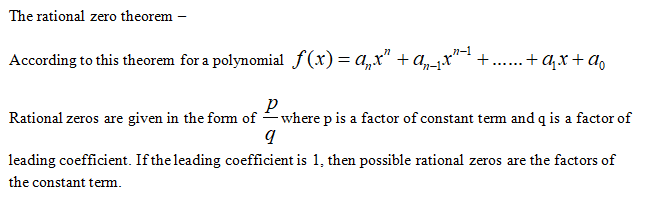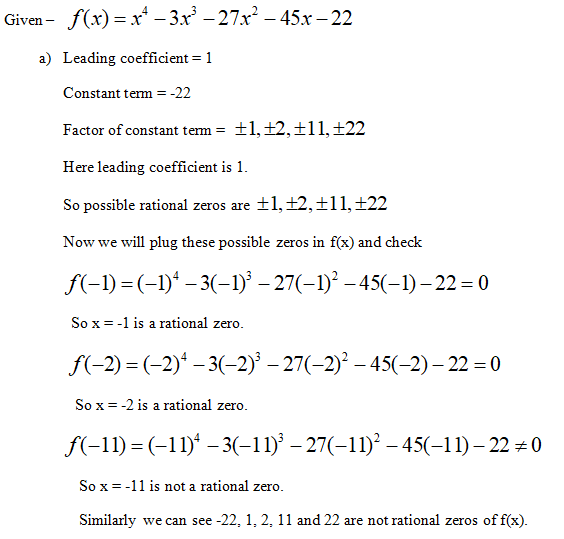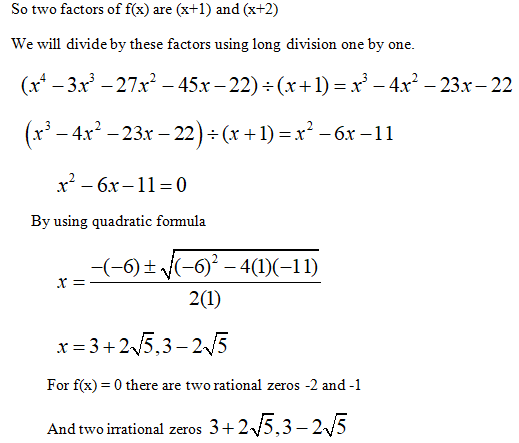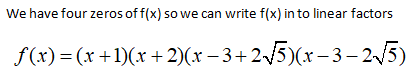# (a) Find the rational zeros and then the other zeros of the polynomial function f(x) = x* - 3x° - 27x - 45x- 22, that is, solve f(x) = 0.(b) Factor f(x) into linear factors.(a) Select the correct choice below and fill in any answer box(es) within your choice.(Use a comma to separate answers as needed. Type an exact answer, using radicals and i as needed.)A. The rational zeros are,and the other zeros areB. There are only rational zeros and they areO C. There are no rational zeros. The other zeros are Find a polynomial of least possible degree having the graph shown.f(x) =(Type your answer in factored form.)5200--10(0,-15825)-15625

Question
4 views

Help

check_circle

Step 1Factor theorem – If c is zero of polynomial f(x) than f(c) =0

Step 2Step 3...

### Want to see the full answer?

See Solution

#### Want to see this answer and more?

Solutions are written by subject experts who are available 24/7. Questions are typically answered within 1 hour.*

See Solution
*Response times may vary by subject and question.
Tagged in